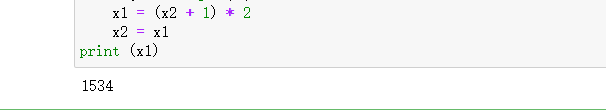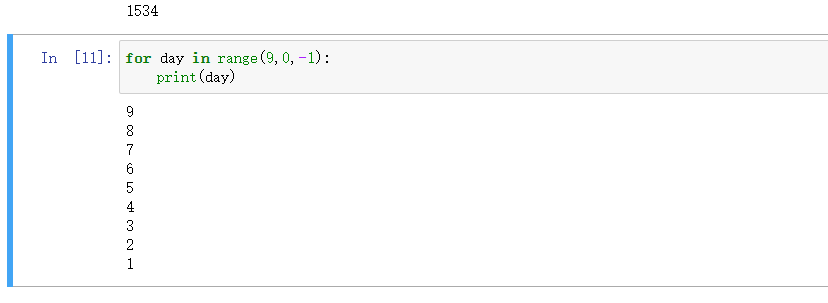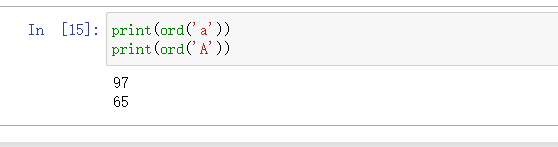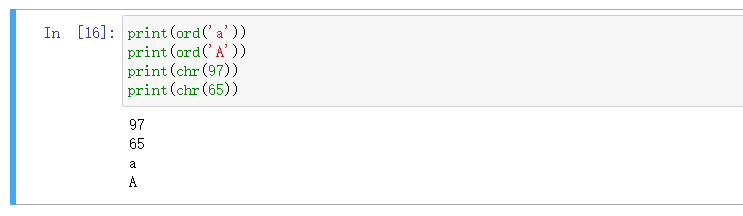﻿ 没有bug队——加贝——Python 练习实例 21，22

### 没有bug队——加贝——Python 练习实例 21，22

2021-11-30 1:01:52

# 21.题目：

``````x2 = 1
for day in range(9,0,-1):
x1 = (x2 + 1) * 2
x2 = x1
print (x1)``````这样应该就理解了吧，嘿嘿🤭

# 22.题目：

``````for i in range(ord('x'),ord('z') + 1):
for j in range(ord('x'),ord('z') + 1):
if i != j:
for k in range(ord('x'),ord('z') + 1):
if (i != k) and (j != k):
if (i != ord('x')) and (k != ord('x')) and (k != ord('z')):
print ('比赛名单是a -- %s\t b -- %s\tc--%s' % (chr(i),chr(j),chr(k)))``````

到这里可能你又有很多问好了吧？

1、中文编码声明注释：# coding=gbk，
2、ord() 函数的作用：获取字符对应的 ASCII 数值，
3、使用 ord() 函数获取字符 a 和 A 的 ASCII 数值，
4、使用 print() 输出获取到的 ASCII 数值

还不理解嘛？到这里我们就知道了，大写的序号要先于小写滴到这里我相信你一定理解了，加油！！